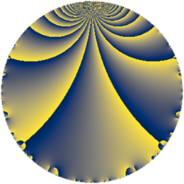# Properties

 Label 7728.2.a.bhLevel $7728$ Weight $2$ Character orbit 7728.a Self dual yes Analytic conductor $61.708$ Analytic rank $1$ Dimension $2$ CM no Inner twists $1$

# Related objects

## Newspace parameters

 Level: $$N$$ $$=$$ $$7728 = 2^{4} \cdot 3 \cdot 7 \cdot 23$$ Weight: $$k$$ $$=$$ $$2$$ Character orbit: $$[\chi]$$ $$=$$ 7728.a (trivial)

## Newform invariants

 Self dual: yes Analytic conductor: $$61.7083906820$$ Analytic rank: $$1$$ Dimension: $$2$$ Coefficient field: $$\Q(\sqrt{33})$$ Defining polynomial: $$x^{2} - x - 8$$ Coefficient ring: $$\Z[a_1, \ldots, a_{5}]$$ Coefficient ring index: $$1$$ Twist minimal: no (minimal twist has level 966) Fricke sign: $$1$$ Sato-Tate group: $\mathrm{SU}(2)$

## $q$-expansion

Coefficients of the $$q$$-expansion are expressed in terms of $$\beta = \frac{1}{2}(1 + \sqrt{33})$$. We also show the integral $$q$$-expansion of the trace form.

 $$f(q)$$ $$=$$ $$q + q^{3} + ( -1 - \beta ) q^{5} + q^{7} + q^{9} +O(q^{10})$$ $$q + q^{3} + ( -1 - \beta ) q^{5} + q^{7} + q^{9} -4 q^{11} + ( -1 - \beta ) q^{13} + ( -1 - \beta ) q^{15} + 2 \beta q^{17} -4 q^{19} + q^{21} + q^{23} + ( 4 + 3 \beta ) q^{25} + q^{27} + ( 7 - \beta ) q^{29} + ( -2 + 2 \beta ) q^{31} -4 q^{33} + ( -1 - \beta ) q^{35} + ( 5 + \beta ) q^{37} + ( -1 - \beta ) q^{39} + ( -1 + 3 \beta ) q^{41} + ( 1 + 3 \beta ) q^{43} + ( -1 - \beta ) q^{45} + ( -7 - \beta ) q^{47} + q^{49} + 2 \beta q^{51} -2 \beta q^{53} + ( 4 + 4 \beta ) q^{55} -4 q^{57} + ( -2 - 2 \beta ) q^{59} -2 q^{61} + q^{63} + ( 9 + 3 \beta ) q^{65} + 4 q^{67} + q^{69} + ( -10 + 2 \beta ) q^{71} + ( -8 + 2 \beta ) q^{73} + ( 4 + 3 \beta ) q^{75} -4 q^{77} + ( 4 - 4 \beta ) q^{79} + q^{81} -4 q^{83} + ( -16 - 4 \beta ) q^{85} + ( 7 - \beta ) q^{87} + ( -2 - 4 \beta ) q^{89} + ( -1 - \beta ) q^{91} + ( -2 + 2 \beta ) q^{93} + ( 4 + 4 \beta ) q^{95} + ( -7 + \beta ) q^{97} -4 q^{99} +O(q^{100})$$ $$\operatorname{Tr}(f)(q)$$ $$=$$ $$2q + 2q^{3} - 3q^{5} + 2q^{7} + 2q^{9} + O(q^{10})$$ $$2q + 2q^{3} - 3q^{5} + 2q^{7} + 2q^{9} - 8q^{11} - 3q^{13} - 3q^{15} + 2q^{17} - 8q^{19} + 2q^{21} + 2q^{23} + 11q^{25} + 2q^{27} + 13q^{29} - 2q^{31} - 8q^{33} - 3q^{35} + 11q^{37} - 3q^{39} + q^{41} + 5q^{43} - 3q^{45} - 15q^{47} + 2q^{49} + 2q^{51} - 2q^{53} + 12q^{55} - 8q^{57} - 6q^{59} - 4q^{61} + 2q^{63} + 21q^{65} + 8q^{67} + 2q^{69} - 18q^{71} - 14q^{73} + 11q^{75} - 8q^{77} + 4q^{79} + 2q^{81} - 8q^{83} - 36q^{85} + 13q^{87} - 8q^{89} - 3q^{91} - 2q^{93} + 12q^{95} - 13q^{97} - 8q^{99} + O(q^{100})$$

## Embeddings

For each embedding $$\iota_m$$ of the coefficient field, the values $$\iota_m(a_n)$$ are shown below.

For more information on an embedded modular form you can click on its label.

Label $$\iota_m(\nu)$$ $$a_{2}$$ $$a_{3}$$ $$a_{4}$$ $$a_{5}$$ $$a_{6}$$ $$a_{7}$$ $$a_{8}$$ $$a_{9}$$ $$a_{10}$$
1.1
 3.37228 −2.37228
0 1.00000 0 −4.37228 0 1.00000 0 1.00000 0
1.2 0 1.00000 0 1.37228 0 1.00000 0 1.00000 0
 $$n$$: e.g. 2-40 or 990-1000 Significant digits: Format: Complex embeddings Normalized embeddings Satake parameters Satake angles

## Atkin-Lehner signs

$$p$$ Sign
$$2$$ $$-1$$
$$3$$ $$-1$$
$$7$$ $$-1$$
$$23$$ $$-1$$

## Inner twists

This newform does not admit any (nontrivial) inner twists.

## Twists

By twisting character orbit
Char Parity Ord Mult Type Twist Min Dim
1.a even 1 1 trivial 7728.2.a.bh 2
4.b odd 2 1 966.2.a.o 2
12.b even 2 1 2898.2.a.x 2
28.d even 2 1 6762.2.a.cd 2

By twisted newform orbit
Twist Min Dim Char Parity Ord Mult Type
966.2.a.o 2 4.b odd 2 1
2898.2.a.x 2 12.b even 2 1
6762.2.a.cd 2 28.d even 2 1
7728.2.a.bh 2 1.a even 1 1 trivial

## Hecke kernels

This newform subspace can be constructed as the intersection of the kernels of the following linear operators acting on $$S_{2}^{\mathrm{new}}(\Gamma_0(7728))$$:

 $$T_{5}^{2} + 3 T_{5} - 6$$ $$T_{11} + 4$$ $$T_{13}^{2} + 3 T_{13} - 6$$ $$T_{17}^{2} - 2 T_{17} - 32$$

## Hecke characteristic polynomials

$p$ $F_p(T)$
$2$ $$T^{2}$$
$3$ $$( -1 + T )^{2}$$
$5$ $$-6 + 3 T + T^{2}$$
$7$ $$( -1 + T )^{2}$$
$11$ $$( 4 + T )^{2}$$
$13$ $$-6 + 3 T + T^{2}$$
$17$ $$-32 - 2 T + T^{2}$$
$19$ $$( 4 + T )^{2}$$
$23$ $$( -1 + T )^{2}$$
$29$ $$34 - 13 T + T^{2}$$
$31$ $$-32 + 2 T + T^{2}$$
$37$ $$22 - 11 T + T^{2}$$
$41$ $$-74 - T + T^{2}$$
$43$ $$-68 - 5 T + T^{2}$$
$47$ $$48 + 15 T + T^{2}$$
$53$ $$-32 + 2 T + T^{2}$$
$59$ $$-24 + 6 T + T^{2}$$
$61$ $$( 2 + T )^{2}$$
$67$ $$( -4 + T )^{2}$$
$71$ $$48 + 18 T + T^{2}$$
$73$ $$16 + 14 T + T^{2}$$
$79$ $$-128 - 4 T + T^{2}$$
$83$ $$( 4 + T )^{2}$$
$89$ $$-116 + 8 T + T^{2}$$
$97$ $$34 + 13 T + T^{2}$$